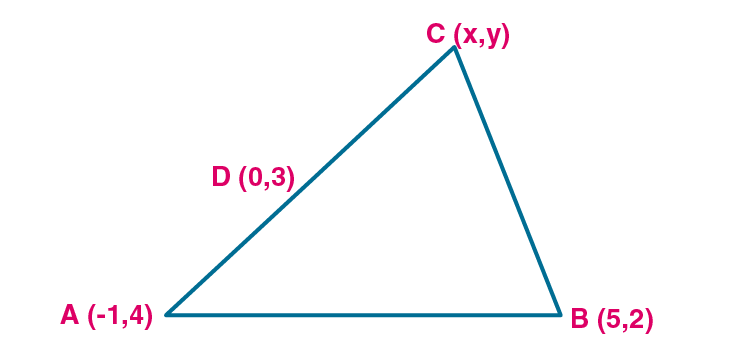Newbie

# Find the third vertex of a triangle if its two vertices are ( – 1, 4) and (5, 2) and midpoint of one sides is (0, 3).

• -2

This is the Important question of class 10 Based on Section Formula Chapter of M.L Aggarwal book for ICSE BOARD.
Here you have to find the third vertex of a triangle if its two vertices are given and midpoint of one sides is also given in the question.
This is the Question Number 27, Exercise 11 of M.L Aggarwal.

Share

1.Let A (-1,4) and B(5,2) are the vertices of the triangle and let D(0,3) is the midpoint of side AC.

Let co-ordinate of C be (x,y).

Consider D(0,3) as midpoint of AC

By midpoint formula,

(-1+x)/2 = 0

-1 +x = 0

x = 1

By midpoint formula,

(4+y)/2 = 3

4+y = 6

y = 6-4 = 2

So the co-ordinates of C are (1,2).

Consider D(0,3) as midpoint of BC

By midpoint formula,

(5+x)/2 = 0

5 +x = 0

x = -5

By midpoint formula,

(2+y)/2 = 3

2+y = 6

y = 6-2 = 4

So the co-ordinates of C are (-5,4).

Hence the co-ordinates of the point C will be (1,2) or (-5,4).

• 2